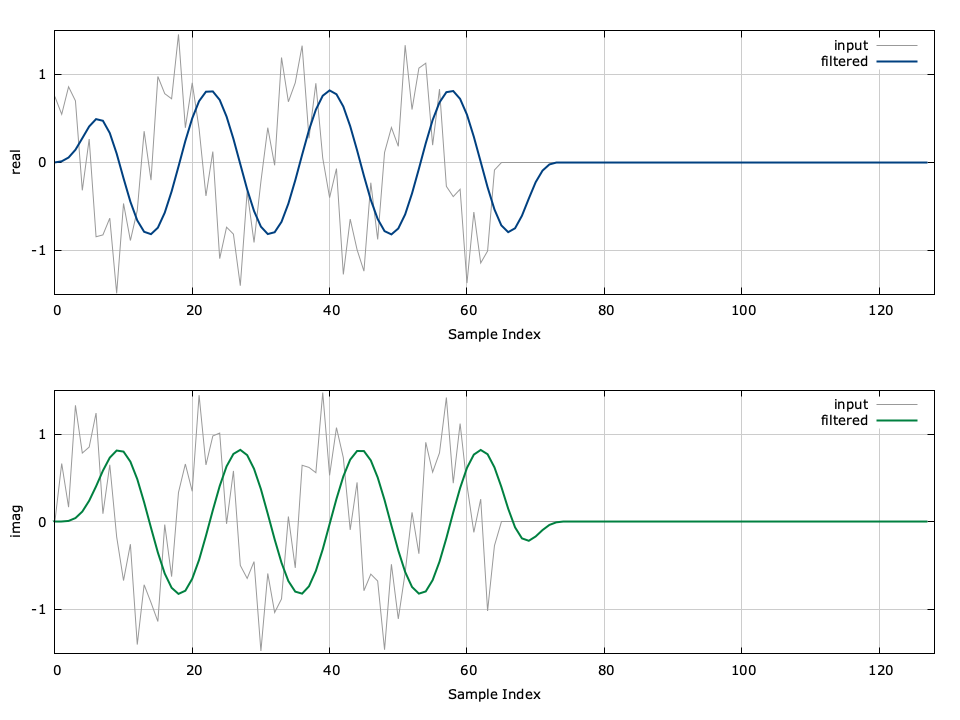# Finite Impulse Response Filter (firfilt)

Finite impulse response (FIR) filters are implemented in liquid with the firfilt family of objects. FIR filters (also known as non-recursive filters ) operate on discrete-time samples, computing the output $$y$$ as the convolution of the input $$\vec{x}$$ with the filter coefficients $$\vec{h}$$ as

$$y(n) = \sum_{k=0}^{N-1}{ h(k) x(N-k-1) }$$

where $$\vec{h} = [h(0),h(1),\ldots,h(N-1)]$$ is the filter impulse response. Notice that the output sample in the above equation is simply the vector dot product (see dotprod ) of the filter coefficients $$\vec{h}$$ with the time-reversed sequence$$\vec{x}$$ .Figure [fig-filter-firfilt_crcf]. firfilt_crcf (finite impulse response filter) demonstration

An example of the firfilt can be seen in[ref:fig-filter-firfilt_crcf] in which a low-pass filter is applied to a signal to remove a high-frequency component. An example of the firfilt interface is listed below.

#include <liquid/liquid.h>

int main() {
// options
unsigned int h_len=21;  // filter order
float h[h_len];         // filter coefficients

// ... initialize filter coefficients ...

// create filter object
firfilt_crcf q = firfilt_crcf_create(h,h_len);

float complex x;    // input sample
float complex y;    // output sample

// execute filter (repeat as necessary)
{
firfilt_crcf_push(q, x);    // push input sample
firfilt_crcf_execute(q,&y); // compute output
}

// destroy filter object
firfilt_crcf_destroy(q);
}


Listed below is the full interface to the firfilt family of objects. While each method is listed for firfilt_crcf , the same functionality applies to firfilt_rrrf and firfilt_cccf .

• firfilt_crcf_create(*h,N) creates a firfilt object with $$N$$ filter coefficients$$\vec{h}$$ .
• firfilt_crcf_recreate(q,*h,N) re-creates a firfilt object $$q$$ with $$N$$ filter coefficients$$\vec{h}$$ ; if the length of the filter doesn't change, the internal state is preserved.
• firfilt_crcf_destroy(q) destroys a firfilt object, freeing all internally-allocated memory.
• firfilt_crcf_print(q) prints the parameters of a firfilt object to the standard output.
• firfilt_crcf_clear(q) clears the internal buffer of a firfilt object.
• firfilt_crcf_push(q,x) pushes an input sample $$x$$ into the internal buffer of the filter object.
• firfilt_crcf_execute(q,*y) generates the output sample $$y$$ by computing the vector dot product (see dotprod ) between the internal filter coefficients and the internal buffer.
• firfilt_crcf_get_length(q) returns the length of the filter.
• firfilt_crcf_freqresponse(q,fc,*H) returns the response of the filter at the frequency $$f_c$$ , stored in the pointer $$H$$ .
• firfilt_crcf_groupdelay(q,fc) returns the group delay of the filter at the frequency $$f_c$$ .